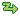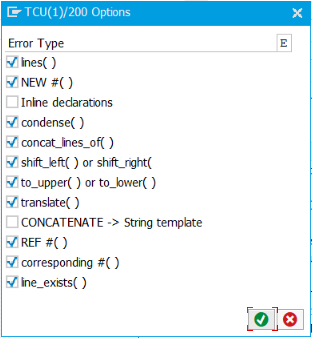# CHECK_45 - Use expressionsRFC enabled
CODE

#### Use lines( ) expression

``````DESCRIBE TABLE lt_table LINES lv_lines.
``````

Can be reduced to

``````lv_lines = lines( lt_table ).
``````

Sometimes `lv_lines` is only used once after this, in this case consider removing the variable and replacing with the expression.

#### Use NEW abc( ) expression

``````DATA: lo_foo TYPE REF TO zcl_foobar.
CREATE OBJECT lo_foo
EXPORTING
iv_moo = 'ABC'.
``````

Can be reduced to

``````DATA(lo_moo) = NEW zcl_foobar( 'ABC' ).
``````

https://github.com/SAP/styleguides/blob/master/clean-abap/CleanABAP.md#prefer-new-to-create-object

#### Declare variable in LOOP statement

``````DATA: ls_foo LIKE LINE OF lt_table.
LOOP AT lt_table INTO ls_foo.
...
ENDLOOP.
``````

Can be reduced to

``````LOOP AT lt_table INTO DATA(ls_foo).
...
ENDLOOP.
``````

Similarily with `FIELD-SYMBOLS`

https://github.com/SAP/styleguides/blob/master/clean-abap/CleanABAP.md#prefer-inline-to-up-front-declarations

#### Use condense( )

``````CONDENSE field.
``````

can be replaced with

``````field = condense( field ).
``````

Since the `NO-GAPS` option of `CONDENSE` is not available for `condense( )`, `replace( )` can be used instead:

``````DATA(my_numbers) = ` 5566551 122 `.

" with CONDENSE
CONDENSE my_numbers NO-GAPS.

" with replace( )
my_numbers = replace( val = my_numbers regex = ` ` with = `` occ = 0 ).

" Result: my_numbers = `5566551122`
``````

#### Use concat_lines_of( )

``````CONCATENATE LINES OF itab INTO field.
``````

can be replaced with

``````field = concat_lines_of( itab ).
``````

#### Use shift_left( ) or shift_right( )

``````SHIFT field LEFT.
``````

can be replaced with

``````field = shift_left( field ).
``````

#### Use to_upper( ) or to_lower( )

``````TRANSLATE field TO UPPER CASE.
``````

can be replaced with

``````field = to_upper( field ).
``````

#### Use translate( )

``````TRANSLATE field USING mask
``````

can be replaced with

``````field = translate( val = field from = from to = to )
``````

#### Use string templates

``````CONCATENATE field1 field2 INTO field3.
``````

can be replaced with

``````field = field1 && field2.
``````

#### Use REF

``````GET REFERENCE OF i_data INTO lo_data.
``````

can be replaced with

``````lo_data = REF #( i_data ).
``````

#### Use CORRESPONDING #( )

``````MOVE-CORRESPONDING struc1 TO struc2.
``````

can be replaced with

``````struc2 = CORRESPONDING #( struc1 ).
``````

Warning: `struc2` will be initialized before the move. On ABAP releases from 740sp08 onwards, the `BASE` addition should be used to avoid this and to keep the statement in line with the functionality of `MOVE-CORRESPONDING`:

``````struc2 = CORRESPONDING #( BASE( struc2 ) struc1 ).
``````

#### Use line_exists( )

``````READ TABLE itab INDEX 1 TRANSPORTING NO FIELDS.
IF sy-subrc = 0.
...
ENDIF.
``````

can be replaced with

``````IF line_exists( itab[ 1 ] ).
...
ENDIF.
``````

Warning: Expression `line_exists` should not be used to first check the existence of row and then read it. Instead, it can be assigned to a field symbol and then `sy-subrc` checked.

### Configuration